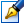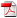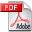##Researching stability in the Lyapunov sense of stationary solutions in the dynamic Albaouy-Grebenicov model (the case of eight planar bodies)

 Author: Cebotaru Elena Degree: doctor of physics and mathematics Speciality: 01.01.09 - Mathematical cybernetics and operation research Year: 2019 Scientific adviser: Evghenii Grebenicovdoctor habilitat, professor Scientific consultant: Mitrofan Ciobandoctor habilitat, professor Institution: Tiraspol State University

### Status

The thesis was presented on the 28 August, 2019
Approved by NCAA on the 27 September, 2019

### Abstract0.97 Mb / in romanian

### Thesis

CZU 519.718, 519.6242.94 Mb / in romanian
127 pages

### Keywords

the restricted problem of n-bodies, mathematical model, central configuration, stationary po int, stabilit y in the first approximat ion, stabilit y in the Lyapuno v sense, Hamiltonians systems, algorithm, computer algebra

### Summary

Thesis structure: the study is written in Romanian and consists of an introduction, 3 chapters, general conclusions, 93 bibliography items, 117 pages of main text, 26 figures and 3 tables. The obtained results were published in 15 scientific papers.

Field of study of the thesis: the theoretical basis, the methods of mathemat ical modeling and analysis of the dynamic models of the celestial mechanics which allow their study with the necessary precision for practical purposes.

The aim of research: The main aim of the paper is to study the influence of the gravitational field of the seven-body configuration on the movement of an infinitely small mass placed in this system. To solve this problem it is necessary to achieve the following object ives: determining the existence conditions of the model, determining the stationary points in the restricted problem, studying the linear stability and Lyapunov stability of the stationary solutions.

Scientific innovation and originality: consists in determining and studying the stabilit y of a new class of solutions in the restricted problem of 7 + 1 bodies whose configuration is a square with two masses on one of the diagonals and the seventh table placed in the origin of the coordinate system coinciding with the center of gravity of the square. Programs have been developed in the Mathematica computing system codes to study the problem.

The main scientific solved problem: consists in the using of the qualitat ive and constructive study methods of the equat ions of the motion of the eight bodies, that describes a mathematical model, which contributed to the determination of the configuration and the existence conditions of the stationary points for their subsequent application in the exact description of the evolution of the dynamic system.

The theoretical significance and applicative value of the thesis: in the current paper it has been shown that there is a square-shaped configuration with two masses on diagonally; it has been demonstrated that there are such dimensions of the configuration for which the stationary points in the restricted problem are stable in the first approximation and Lyapunov sense.

The implementation of the scientific results: The algorithms and computer programs developed in the thesis have made it possible to effectively determine the model's existence conditions and to study the stability of stationary solutions. They can be used in the study of other mathemat ical models of celest ial mechanics. The results of the thesis can be used in the design, management of space flights, in the teaching of different ial equations, celestial mechanics, stability theory, mathematical modeling methods.